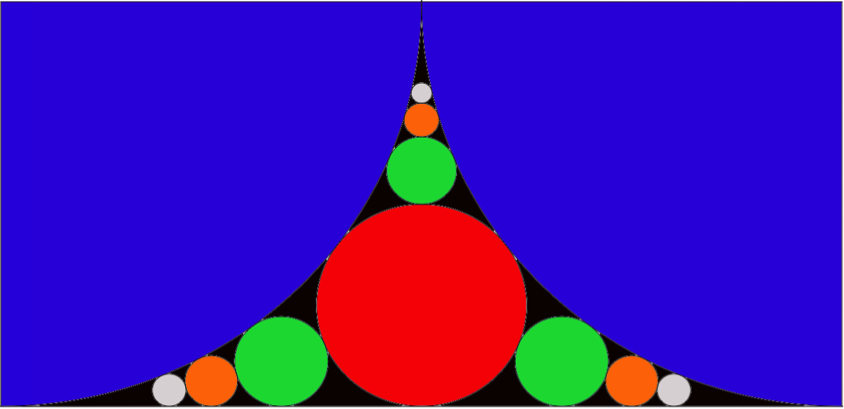# Revival of Sangaku

Geometry Level 5The radius of the blue circular quadrants is one. If the above pattern continues ad infinitum, express the area of the nested circles as an infinite sum. Once that is done, calculate the total area of the nested circles.

Notes:

1) As illustrated above, the pattern expands in three directions only (up - up, left - left, right - right)

2) The nested circles do not include the two blue quadrants

×